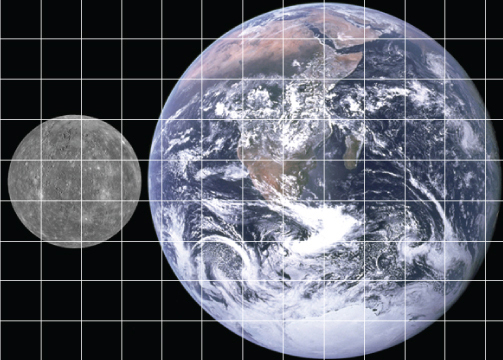An introduction to exoplanets

Start this free course now. Just create an account and sign in. Enrol and complete the course for a free statement of participation or digital badge if available.

Free course

# Comparing areas for circles of differing size

In Week 2 you learned that the volume of a sphere depends on the radius cubed (radius × radius × radius, that is, r3). There you saw that increasing the radius by a factor of 10 increases the volume by a factor of 10 × 10 × 10 = 1000.

The area of a circle depends on the radius squared (radius × radius, that is, r2). In a similar way, if the radius is increased by a factor of 10 then the area is increased by a factor of 10 × 10 = 100.

Similarly, if the radius is doubled, the area is increased by a factor of four. The factor of four comes about because the radius is multiplied by itself, and 2 × 2 = 4.

The Earth’s radius is close to three times that of Mercury’s. Figure 14 shows how much larger the Earth’s cross-sectional area is than Mercury’s: it’s a bit less than 3 × 3 = 9 times the area. Count the squares overlying each planet to verify this.Figure 14  Cross-sectional areas of Mercury and Earth

## Activity 2  Cross-sectional areas

You have been given the approximate radii of the Sun and some of the Solar System planets. You need to select the correct ratio of the cross-sectional areas between two objects from a list of possible answers. Use a calculator if you need to. (Remember that RE represents the radius of Earth.)

Sun: approximately 100 RE

Jupiter: approximately 10 RE

Mercury: approximately 0.5 RE

Venus: approximately 1 RE

In the rest of this activity ‘area’ refers to the cross-sectional area.

1. Is the area of the Sun:

a.

10 times the area of Jupiter?

b.

100 times the area of Jupiter?

c.

20 times the area of Jupiter?

The radius of the Sun is 10 times the radius of Jupiter so the area of the Sun is 10 × 10 = 100 times the area of Jupiter.

2. Is the area of Venus:

a.

1/100th the area of the Sun?

b.

1/1000th the area of the Sun?

c.

1/10 000th the area of the Sun?

The radius of Venus is th the radius of the Sun so the area of the Venus is th the area of the Sun.

(Alternatively, the radius of the Sun is 100 times the radius of Venus so the area of the Sun is 100 × 100 = 10 000 times the area of Venus, and hence the area of the Venus is th the area of the Sun.)

3. Is the area of Jupiter:

a.

400 times the area of Mercury?

b.

20 times the area of Mercury?

c.

40 times the area of Mercury?

The radius of Jupiter is 10 times the radius of Earth, and the radius of Earth is two times the radius of Mercury. This means that the radius of Jupiter is 20 times the radius of Mercury. Therefore, the area of Jupiter is 20 × 20 = 400 times the area of Mercury.

EXO_1